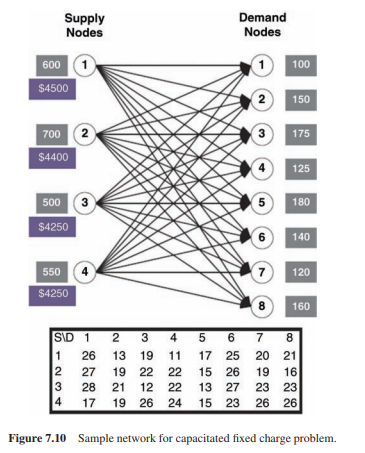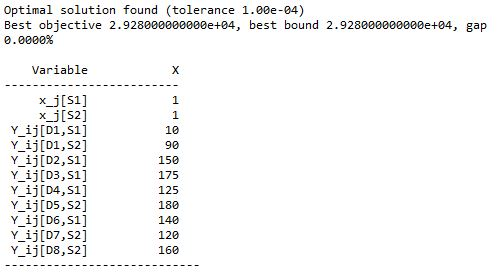•Gurobi Staff

Hi Ankit,

Could you please edit your question and properly format your code? See Posting to the Community Forum for help on how to do that.

And please try summarizing the output - currently, this is just a long wall of text and your actual question is hard to understand.

Thanks,
Matthias

•Dear sir,
I have modified the question as suggested.

Thanks
Ankit

•Gurobi Staff

Hi Ankit,

I recommend testing your code on a small toy problem so you can inspect and analyze every single step.

One thing I noticed is that you are using a pretty old Gurobi version. You should definitely update to the latest version to get all improvements and bug fixes developed in the meantime.

Best regards,
Matthias

•Dear sir,

Thankyou very much for the reply.

I have tried to run the code with updated version Gurobi 9.5, still I am getting the same results.

We are solving a very small example problem on "Capacitated Fixed Charge problem" given in book of "Network and Discrete Location" by Daskin in page number 331.We have modelled this problem in Python and solved it using Gurobi 9.5. The code is given below. We are getting an optimal solution in seconds.

import gurobipy as gpfrom gurobipy import*Supply_nodes = ['S1', 'S2', 'S3', 'S4']Demand_nodes = ['D1', 'D2','D3','D4','D5','D6','D7','D8']Fixed_cost = {'S1':4500, 'S2':4400, 'S3':4250, 'S4':4250}Capacity = {'S1':600, 'S2':700, 'S3':500, 'S4':550}Demand = {'D1':100, 'D2':150, 'D3':175, 'D4':125, 'D5':180, 'D6':140, 'D7':120, 'D8':160}Transport_input = [26, 27, 28, 17, 13, 19, 21, 19, 19, 22, 12, 26, 11, 22, 22, 24, 17, 15, 13, 15, 25, 26, 27, 23, 20, 19, 23, 26, 21, 16, 23, 26]Transport_cost = {}X = 0for i in Demand_nodes:for j in Supply_nodes:Transport_cost[(i,j)] = Transport_input[X]X = X + 1m=gp.Model()x_j = m.addVars(Supply_nodes,vtype=GRB.BINARY,name='x_j')y_ij = m.addVars(Demand_nodes, Supply_nodes, vtype=GRB.CONTINUOUS,name='Y_ij')m.setObjective(sum(Fixed_cost[j]*x_j[j] for j in Supply_nodes) + sum(Transport_cost[i,j]*y_ij[i,j] for i in Demand_nodes for j in Supply_nodes))for i in Demand_nodes:m.addConstr(sum(y_ij[i,j] for j in Supply_nodes) == Demand[i])for j in Supply_nodes:m.addConstr(sum(y_ij[i,j] for i in Demand_nodes) <= Capacity[j]*x_j[j])m.optimize() m.printAttr('X')

After running this code, we are getting an optimal solution i.e. 29280.Now, we are trying to solve the same problem with the benders decomposition using callback.
which is discussed in next thread.

Regards
Ankit

•Dear sir

We are trying to solve a very small example  "Capacitated Fixed Charge problem" (discussed in earlier thread) using Benders decomposition.

We have decomposed the problem in Master problem and Sub-problem.

Master problem----->>

##### Master problem ##########mp = gp.Model()x_j_mp = mp.addVars(Supply_nodes,vtype=GRB.BINARY,name='x_j_mp')D = mp.addVar(vtype=GRB.CONTINUOUS,name='D')mp.setObjective(sum(Fixed_cost[j]*x_j_mp[j] for j in Supply_nodes) + D)mp.addConstr(sum(Capacity[j]*x_j_mp[j] for j in Supply_nodes) >= sum(Demand[i] for i in Demand_nodes))mp._vars = x_j_mp

Sub problem----->>

##### Sub problem ##########sp = gp.Model()U_i = sp.addVars(Demand_nodes, lb=-1e20, ub=1e20, vtype=GRB.CONTINUOUS,name='U_i')W_j = sp.addVars(Supply_nodes, vtype=GRB.CONTINUOUS, name='W_j')for i in Demand_nodes:    for j in Supply_nodes:        sp.addConstr( U_i[i] - W_j[j] <= Transport_cost[i,j])

Further, we are using callback function for inserting Lazy constraint.

LB = 0 UB = 1000000000 UB_temp = 0 X_val = {} U_val = {} W_val = {}Obj_list= [] bound_list = [] iteration = 0 global iterNum iterNum = 0def mycallback(model, where):     if where == GRB.Callback.MIPSOL:       print("****MIP sol callback*****")       global iterNum       print ("Going for BD iter ", iterNum)       # nodecnt = mp.cbGet(GRB.Callback.MIPSOL_NODCNT )       obj = model.cbGet(GRB.Callback.MIPSOL_OBJ)       bound = model.cbGet(GRB.Callback.MIPSOL_OBJBND)       print("Best known LOWER bound = ", bound)       print("****************************************")       print("OBJ VALUE = ", obj)       print("bound = ",bound)       print("****************************************")       Obj_list.append(round(obj))       bound_list.append(bound)       vars_val = {}       vars_val = model.cbGetSolution(model._vars)       print(vars_val)       D_val = 0       D_val = model.cbGetSolution(D)       print("D var value = ", D_val) print(X_val)       for j in Supply_nodes:           X_val[j] = vars_val[j]           if X_val[j] == 1:                print("Open = ", j)       print(X_val)       print("SP SOl------>>>>>")       sp.setObjective(sum(Demand[i]*U_i[i] for i in Demand_nodes) - sum(Capacity[j]*X_val[j]*W_j[j] for j in Supply_nodes), GRB.MAXIMIZE)       sp.update()       sp.optimize()       for i in Demand_nodes:           U_val[i] = U_i[i].x       for j in Supply_nodes:           W_val[j] = W_j[j].x       UB_temp = 0       UB_temp = sum(Fixed_cost[j]*X_val[j] for j in Supply_nodes) + sp.ObjVal       print("UB Temp = ", UB_temp)       D_RHS = 0       D_RHS = (sum(Demand[i]*U_val[i] for i in Demand_nodes) - sum(Capacity[j]*X_val[j]*W_val[j] for j in Supply_nodes))       print("D var value = ", D_val)       print("D_RHS = ", D_RHS)       if D_val < D_RHS:           model.cbLazy(D >= sum(Demand[i]*U_val[i] for i in Demand_nodes) - sum(Capacity[j]*model._vars[j]*W_val[j] for j in Supply_nodes))        iterNum = iterNum + 1mp.Params.lazyConstraints = 1 mp.Params.PreCrush = 1mp.Params.Threads = 1 mp.optimize(mycallback)print(time.time() - t0)print("----------------------------")print("----------------------------")mp.printAttr('X')print("Obj list-->>",Obj_list)print("Bound list--->>",bound_list)

After running this code, we are getting an optimal solution i.e. 29280 which is same as what we were getting in original formulation.

The value of upper bound and lower bound which we are getting in each iteration is given below.

Obj list-->> [8650, 8650, 8900, 8900, 23880, 31445, 27845, 28450, 30560, 30074, 30246, 28450, 29870, 27860, 29430, 29280]Bound list--->> [-1e+100, -1e+100, -1e+100, 0.0, 20880.0, 20880.0, 20880.0, 25008.7926540619, 25008.7926540619, 25342.559303459075, 25841.913611781845, 26951.888466413187, 26951.888466413187, 27143.500000000004, 27143.500000000007, 27605.000000000004]

Now, my query is

For the minimization problem, the obj value (UB) should be decreasing continuously in each iteration, but this is not the case here. As we can see in the Obj list, the obj value is not decreasing continuously, but fluctuating.

Sir, I have analyzed each iteration of benders, still I am not able validate that my callback implementation is correct due to the unusual behavior of UB.

This is my request that please validate whether I am implementing callback correctly or not.
And please suggest if this behavior is common or we are making some mistakes during implementation.

The code and the log file of the solution is attached through dropbox.

We will be very grateful to you for your response.

Thanks and Regards
Ankit Chouksey